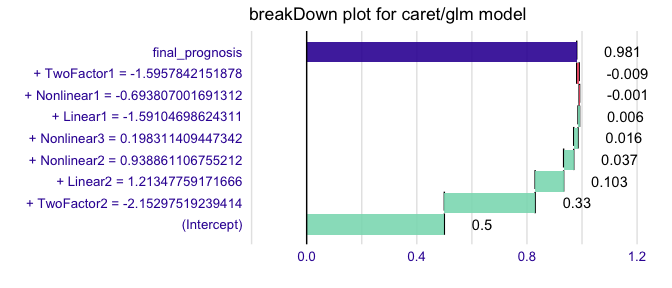# How to use breakDown package for models created with caret

#### 2020-04-04

This example demonstrates how to use the breakDown package for models created with the caret package.

First we will generate some data.

library(caret)

set.seed(2)
training <- twoClassSim(50, linearVars = 2)
trainX <- training[, -ncol(training)]
trainY <- training\$Class

#>   TwoFactor1 TwoFactor2    Linear1    Linear2 Nonlinear1 Nonlinear2 Nonlinear3
#> 1 -0.6561702 -1.6480450  1.0744594  0.9758906  0.2342843  0.6805653  0.6920055
#> 2 -0.9849973  1.4598834  0.2605978 -0.1694232  0.1381283  0.7460168  0.5599569
#> 3  2.3722541  1.7069944 -0.3142720  0.7221918 -0.6920591  0.4642024  0.3426912
#> 4 -2.2067173 -0.6972704 -0.7496301 -0.8444186 -0.9303336  0.1374181  0.2344975
#> 5  0.5166671 -0.7228376 -0.8621983  1.2772937  0.9959069  0.8143796  0.4296028
#> 6  1.3331262 -0.9929323  2.0480403 -1.3431105  0.6711474  0.8321613  0.7367007
#>    Class
#> 1 Class1
#> 2 Class2
#> 3 Class1
#> 4 Class2
#> 5 Class1
#> 6 Class1

Now we are ready to train a model. Let’s train a glm model with caret.

cctrl1 <- trainControl(method = "cv", number = 3, returnResamp = "all",
classProbs = TRUE,
summaryFunction = twoClassSummary)

test_class_cv_model <- train(trainX, trainY,
method = "glm",
trControl = cctrl1,
metric = "ROC",
preProc = c("center", "scale"))
test_class_cv_model
#> Generalized Linear Model
#>
#> 50 samples
#>  7 predictor
#>  2 classes: 'Class1', 'Class2'
#>
#> Pre-processing: centered (7), scaled (7)
#> Resampling: Cross-Validated (3 fold)
#> Summary of sample sizes: 33, 34, 33
#> Resampling results:
#>
#>   ROC        Sens       Spec
#>   0.7771991  0.7175926  0.8009259

To use breakDown we need a function that will calculate scores/predictions for a single observation. By default the predict() function returns predicted class.

So we are adding type = "prob" argument to get scores. And since there will be two scores for each observarion we need to extract one of them.

predict.fun <- function(model, x) predict(model, x, type = "prob")[,1]
testing <- twoClassSim(10, linearVars = 2)
predict.fun(test_class_cv_model, testing[1,])
#>  0.9807632

Now we are ready to call the broken() function.

library("breakDown")
explain_2 <- broken(test_class_cv_model, testing[1,], data = trainX, predict.function = predict.fun)
explain_2
#>                                   contribution
#> (Intercept)                              0.500
#> + TwoFactor2 = -2.15297519239414         0.330
#> + Linear2 = 1.21347759171666             0.103
#> + Nonlinear2 = 0.938861106755212         0.037
#> + Nonlinear3 = 0.198311409447342         0.016
#> + Linear1 = -1.59104698624311            0.006
#> + Nonlinear1 = -0.693807001691312       -0.001
#> + TwoFactor1 = -1.5957842151878         -0.009
#> final_prognosis                          0.981
#> baseline:  0

And plot it.

library(ggplot2)
plot(explain_2) + ggtitle("breakDown plot for caret/glm model")